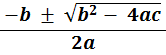### Solving quadratics of the form x2 + bx + c = 0 − using the quadratic formula

Solving quadratics of the form ax2 + bx + c = 0 using the quadratic formula:

 x =In the examples that follow a is always equal to 1

• #### Example 1.   Solve x2 + 7x + 6 = 0

• x2 + 7x + 6 = 0           here a = 1, b = 7 and c = 6

Verify by putting these two values of x into x2 + 7x + 6

• #### Example 2.   Solve x2 − 11x + 30 = 0

• x2 − 11x + 30 = 0           here a = 1, b = − 11 and c = 30

Verify by putting these two values of x into x2 − 11x + 30

• #### Example 3.   Solve x2 + 2x − 8 = 0

• x2 + 2x − 8 = 0           here a = 1, b = 2 and c = − 8

Verify by putting these two values of x into x2 + 2x − 8

• #### Example 4.   Solve x2 − 5x − 24 = 0

• x2 − 5x − 24 = 0           here a = 1, b = − 5 and c = − 24

Verify by putting these two values of x into x2 − 5x − 24to: